# Differential ionosphere modelling for single-reference long-baseline GPS kinematic positioning

## Abstract

The ionospheric effect is considered to be one of the most important error sources limiting the quality of GPS kinematic positioning. Over longer distances, differential ionospheric residuals become larger and may affect the ambiguity resolution process. We present here a Kalman-filter-based GPS ionosphere model for long-baseline kinematic applications. This observational model includes the differential ionosphere as an additional unknown factor with position coordinates and ambiguities, while the temporal correlations of the state vector are specified in the dynamic model. The temporal behaviour of ionospheric residuals is determined by the analysis of their autocorrelation function. This newly developed method was applied on a set of data collected by a roving receiver located offshore of Oran (Algeria). The results show that for baselines of about 80 km, the root mean square is at the level of a few centimetres. For tests of baselines of about 51 km, the comparison between short- and longbaseline solutions revealed that mean differences of a few millimetres and 2 cm are obtained for the horizontal coordinates and vertical component, respectively, and the standard deviation (σ) of differences on the scale of a few centimetres.

## 1. Introduction

The development of the GPS kinematic technique has enabled real-time accurate positioning of a mobile platform to be performed. For such accurate GPS kinematic positioning, however, it is necessary to determine the integer number of carrier phase cycles, which is called “integer ambiguities”. Over short baselines, the double-difference (DD) technique can be applied to cancel out most of the correlated errors. Another option—and a common practice—is simply to disregard their effects. However, in the case of long-baseline kinematic positioning, the ionospheric effect become increasingly more decorrelated, and it may affect the ambiguity resolution process, or even make it impossible to compute (Vollath et al., 2000; Wielgosz et al., 2005). Therefore, reducing the differential ionospheric effect is one of the most important steps towards improving ambiguity resolution and to achieve accurate medium- and long-range kinematic positioning (Odijk, 2000; Vollath et al., 2000; Kashani et al., 2005; Wielgosz et al., 2005). In recent years, many approaches have been developed to enable high-accuracy GPS kinematic positioning over longer distances (Wübbena et al., 1996; Han, 1997; Raquet, 1998; Wanninger, 1999; Lachapelle et al., 2000; Odijk et al., 2000; Hernandez-Pajares et al., 2000; Cannon et al., 2001; Rizos, 2002; Hu et al., 2003; Chen et al., 2004; Wielgosz et al., 2004, 2005). All of these investigations involve the use of multi-reference stations. However, a network of GPS reference stations is not always available and its implementation is costly. In addition, in certain cases (e.g., marine long-baseline applications), interpolated corrections are not reliable because the rover receiver is usually outside the network coverage area. A new approach based on a single-reference-station mode has been published by Kim and Langley (2007) that nullifies the effect of the differential ionosphere in an ambiguity search process; this method provides a number of interesting results.

In this paper, GPS kinematic positioning is implemented in a single-reference-station mode. Our approach is based on the use of the Kalman filtering method to estimate the differential ionospheric delay as a state with unknown position coordinates and ambiguities. To model the ionospheric delays as a state, an additional parameter for each satellite must be included in the observation equations that involves a singularity of the equation system (i.e., the number of unknown parameters becomes greater than the number of observations). To overcome this problem, the redundancy is increased by using dual-frequency carrier phases (L1 and L2) and code pseudo-range (P1, P2 and C/A) measurements.

The Kalman filtering method is based on the use of two models: the observation model (for updating or correction) and the underlying dynamic model (for prediction). The observation model describes the relationship between the observations and unknown parameters, while the dynamic model allows the user to predict the state parameters from a previous time step. The dynamic model describes the time-dependent relationship between successive values of the same state. The ionospheric effect is usually considered to be a time-dependent signal that continuously changes in time as the electron density varies with time and location in the ionosphere (Coco, 1991; Klobuchar, 1991; Ming, 1999). To characterise the temporal behaviour of the ionosphere, we have calculated and analysed the autocorrelation of phase DD ionospheric residuals and thereby obtained the dynamic model. The developed method was applied, with success, on a set of data collected by a roving receiver offshore Oran (Algeria).

## 2. Methodology

### 2.1 GPS observation equations

The GPS dual frequency DD observables measured between r and m (subscript) receivers, and satellites i and j (superscript) are given as follows:(1)

In Eq. (1), ϕ1, ϕ2 and P 1 , P 2 are the phase ranges and pseudo-ranges measured at the L1 and L2 frequencies, respectively. The term ρ is the geometric distance between the two satellite antennas and the two receiver antennas, while I/f2 stands for the first-order ionosphere refraction. The wavelengths of the L1 and L2 phases are γ1 ≈ 19 cm and γ2 ≈ 24 cm, respectively. The tropospheric refraction is T, and the measurement noise is characterised by the error terms ε and e. The integer ambiguities associated with the L1 and L2 phases are denoted as N 1 and N2, respectively. Multipath and receiver antenna phase centre variations are ignored in Eq. (1). One can combine the geometric distance p and the tropospheric delay T to obtain the ideal pseudorange as:(2)

In this study, the Goad and Goodman model is used to estimate tropospheric delay. Actually, several tropospheric models may be applied, of which are those of Essen and Froome (1951), Hopfield (1969), Saastamoinen (1972), Goad and Goodman (1974), and Chao (1974). When the elevation angle is >20°, different models give very similar estimates of the tropospheric refraction (Ho, 1990; Rothacher, 1992; Solheim, 1993).

The linearization of Eq. (1) gives:(3)

in which Z is the observed-minus-computed vector for the four measurements. The error vector is V, and H is known as the design matrix. The state vector used in this study is given, for one epoch, as:(4)

where; represent the corrections made to the approximate receiver position. The term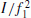stands for the L1 DD ionospheric residual. N1 and N1 — N2 are the DD ambiguity integers of the L1 and L1 — L2.

### 2.2 Ionosphere modelling

In order to stochastically analyse the ionospheric residuals, we collected and used test data from a base-receiver situated in the harbour of Oran and data from a rover antenna embedded on a 9-m-long boat located offshore Oran. Data were recorded by two Ashtech-Z12 GPS receivers during the morning (0800—1000 hours Local Time) of June 17, 2002, with a sampling rate of 01 s. The analysis is based on the calculation of the autocorrelation function of geometry-free DD measurements (DD ionospheric residuals) for the selected receiver-satellite pairs. All the DD pairs are based on the reference-rover baseline, which varies from 100 m to 80 km, and two sets of satellite pairs (PRN 02-03, and PRN 02-31) were chosen for this analysis.

#### 2.2.1 DD ionospheric residuals:

The correlated nature of the ionosphere allows the major part of its effect to be eliminated by forming the DD phase observables. Nevertheless, when baselines are longer than few tens of kilometres, residual errors still remain.

The ionospheric residuals I may be obtained by forming the so called geometry-free linear combination of L1 and L2 phase measurements (Xinhua, 1992).(5)

Figures 1 and 2 show the differential ionosphere effect on the L1 phase observable for the satellite pairs 02–03 and 02–31, respectively. A centimetre level is observed for a baseline of 40 km; the level tends to increase as the distance between the base receiver and the rover increases.

#### 2.2.2 Analysis of the autocorrelation of DD ionospheric residuals:

In order to model the differential ionosphere, its autocorrelation function is analysed. From the results of this analysis, it is possible to model the ionospheric residuals as a first-order Gauss-Markov process.

In general, a first-order Gauss-Markov process can be expressed in the following differential form (Zhang, 1999):(6)

whereis the correlation time,is the perturbation (a zero-mean white noise of variance, and I represents the DD ionospheric residuals.(7)

where E [ ] denotes the expectation operator, andis the Dirac delta function.

The discrete solution of Eq. (6) may be written as:(8)

where the parameter ϕ is given by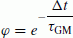(9)

which is a measurement of the exponential correlation between adjacent measurements of sampling interval Δt. The autocorrelation function of a first-order Gauss-Markov process is given by Zhang (1999):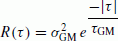(10)

In Fig. 3, the typical autocorrelation function plot of a first-order Gauss-Markov random process is shown.

Figures 4 and 5 describe the normalised autocorrelation of the DD ionospheric residuals for the selected satellite pairs. Comparing these plots with the typical plot of the autocorrelation of first-order Gauss-Markov processes (Fig. 3), it is reasonable to consider that the DD ionospheric residuals behave as first-order Gauss-Markov processes because their autocorrelation functions show a clear exponential attenuation trend similar to that of Fig. 3.

### 2.3 Kalman filter

A Kalman filter algorithm is used to estimate the system state vector X, which is grouped as follows:(11)

The prediction (—) of the state vector at the next epoch n +1 is derived from the update (+) of epoch n as:(12)

The parameter Xp in Eq. (11) contains the three-dimensional position coordinates, the velocity, and the acceleration of the moving receiver.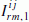is the residual DD ionosphere. The remaining parameters refer to L1 and wide-lane (L1—L2) ambiguities. Each group is treated independently, i.e., there are no correlations between the groups. Therefore, the state transition matrix ϕ and the process noise covariance matrix W are in block diagonal forms:(13)(14)

Position, velocity and acceleration vectors Xp are expressed in the local topocentric northing, easting and height coordinate system. We ignore any inter-correlation between each component, thus treating each of these as independent of each other. Also, similar to the majority of vehicle motion, the modelling of position and velocity in each dimension (second order system) is considered.

A second-order system equation in one dimension is (Leick, 1995)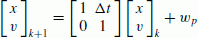(15)

in which x is the position, v is the velocity, ϕt is the observation period, and(16)

The symbol q p denotes variance of the process noise.

In summary, the state vector X p , the transition matrix ϕ p and the process noise matrix Wp for a typical dynamic system, in the northing, easting, and height coordinate system, are(17)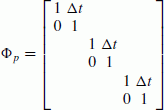(18)(19)

in which (x, y, z) are the vector components of northing, easting, and height, respectively, in the geodetic horizon plane at the fixed station, (vx, vy, vz) are the respective velocity components, and (qx qy qz) are the variance of the process noise in the same components. All other empty entries are zero.

For DD ionospheric residuals, the state vector, the transition matrix, and the process noise matrix for n double-differences are: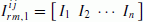(20)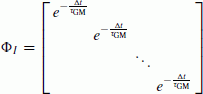(21)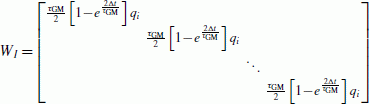(22)

with q i being the variance of the residual ionosphere process noise for the correlation time.

The DD integer ambiguities are simply modeled as constants. The states are transformed into L1 integer ambiguities and wide-lane integer ambiguities to take advantage of the longer wide-lane wavelength (86 cm).

### 2.4 DD ambiguity resolution

To achieve a GPS kinematic position on the centimetre scale, it is necessary to resolve the integer ambiguities in the carrier phase measurements. Float ambiguities are estimated as part of the state vector with a Kalman filtering algorithm. The DD integer ambiguities are modelled as constants and are treated as independent from each other.

After an initial transition period and when the estimated integer ambiguities variances reach a predetermined criteria, an integer ambiguity search is launched using estimated values as initial guesses. Ambiguities will be fixed to integers when the ambiguity search criteria is met.

The search procedure consists in testing all combinations included in a search space and to determine which combination minimises the following quadratic form:(23)

whereis the float ambiguities vector,is the integer ambiguities vector,is the float ambiguities variance-covariance matrix.

To take advantage of the longer wide-lane wavelength (≈86 cm), we first fix the wide-lane integer ambiguities, following which it is relatively easy to determine the L1 integer ambiguities.

## 3. Test Results and Analysis

The method described here relies on the use of DD phase and code measurements, and interest in its application resides in the elimination of satellite and receiver clock biases and the reduction of spatially correlated errors, such as orbit error and atmospheric effects. For a reliable ambiguity resolution, the wide-lane combination was used as it enables the number of candidates included in the research space to be reduced, and this combination is less affected (in cycle) by the set of errors affecting the phase measurements. In order to reduce the effect of the multipath, as well as the atmospheric effect, we used an elevation mask of 15°. The small jumps in cycles are practically unavoidable and are due to the conditions under which the survey was performed (agitated or quiet sea).

In the Kalman filtering method, the dynamic model should be correctly specified. In our study, horizontal coordinates are modelled as an integrated velocity, while the average vertical component is modelled as a constant. For differential ionosphere modelling, the autocorrelation analysis of the DD ionospheric residuals, performed previously, shows that ionospheric residuals can be modelled as a firstorder Gauss-Markov random process.

Several alternatives have been considered in modelling the correlation time of the ionospheric residuals, including the white noise model (WN), Gauss-Markov process, and the random walk model.

Figure 6 shows the averaged post-fit phase residuals for several data processing cases, which are shown in Table 1. In the first case (N/A), we assume that ionospheric residuals are zero. In the second case (WN), they are modelled as being statistically time independent at each epoch (the correlation time is set at zero); the process noise of this WN model was set at 1.0 ppm of the baseline length. In the third case, the temporal behaviour of ionospheric residuals is modelled as a Gauss-Markov process; the correlation time was set at 100 s, the process noise at 0.5 ppm of the baseline length, and the initial uncertainty of the ionosphere at 1.5 ppm of the receiver separation. In the fourth case, we consider a random walk model.

According to Fig. 6, the correlation time value that minimises the averaged post-fit phase residuals is 100 s.

To quantify the position error induced by bad modelling of the differential ionosphere, the Gauss-Markov model (with 100 s of correlation time) is taken as a reference because residuals are minimum. Rover position coordinates are calculated for the four previous cases. Figure 7 shows the ship’s trajectory; rover positions are determined a 1-s intervals and are expressed in a local topocentric coordinate system for which the reference station is the origin. The observed baselines vary from some hundreds of metres to 80 km.

Figures 8 and 9 show the difference in the horizontal East (x) and the height (z) component, respectively. Differences are calculated between the third case and the other remaining cases described in Table 1. The non-modelling of ionospheric residuals introduces a maximal error of 28 cm in the x component and 25 cm in the z component. At the beginning of observations, differences of about 60 cm correspond to the initial transition period, where ambiguities are not yet resolved.

For distances of about 40 km, the random walk model gives similar results to those obtained for the Gauss-Markov model (with a correlation time of 100 s). This may be discerned from Figs. 8 and 9 where the differences in x and z fluctuate around zero, until the time tag 32.75 (which corresponds to a baseline of about 40 km).

Figure 10 shows the time behaviour of the geometric dilution of precision (PDOP) and the number of satellites tracked during the surveys; the abrupt changes in the PDOP were caused by the temporary loss of the signal on some satellites. Figure 11 shows the time variation of the estimated values of the root mean square (RMS) positions (obtained for the Gauss-Markov model with a correlation time of 100 s). As indicated, the RMS has a sharp transition between time tag 30.5 and 31, which indicates that the DD integer ambiguities were determined. The estimated RMS position varies from 3 to 10 cm.

A second set of data recorded (sampling rate 1 s) at a pair of base stations (DT003 and DT004) and with a roving receiver placed on a boat on 13 July 2009 is used. The rover receiver was located a few hundred metres from the DT004 station and about 51 km distant from the DT003 station. Consequently, the data provided both short and long baselines at the same time. Figure 12 shows the location of the rover and the two base stations.

To validate our approach, we compared the long- and short-baseline solutions. The short-baseline solution is used as reference since the all of the systematic errors, those on the ionospheric effect in particular, are correlated and therefore eliminated by differencing.

The right side of Fig. 13 shows that the long- (DT003 to boat) and short- (DT004 to boat) baseline solutions differ by only a few centimetres in terms of local geodetic coordinates when the ionospheric residuals are estimated. These differences are caused by the presence of the multipath and the tropospheric residuals, which are more significant on the vertical component (about 6 cm for certain epochs). Table 2 summarises the statistics. Mean differences of a few millimetres are obtained for the horizontal coordinates and a difference of 2 cm if obtained for the vertical component; the standard deviation (Std; σ) of differences is at a level of 1–2 cm only. Without modelling the ionosphere, differences may achieve the decimetre level (see left side of Fig. 13).

Figure 14 shows that the ambiguities are fixed after less than 3 min. After the initialisation (fixing ambiguities), the RMS reach the centimetre level.

## 4. Conclusion

In the study reported here, we have used the single-reference GPS kinematic positioning method to accurately determine the trajectory of a ship in the water offshore of Oran (Algeria).

In kinematic positioning, the ionospheric effect is the main source of error affecting the integer ambiguity resolution process and, consequently, the position accuracy. The ionospheric effect becomes increasingly decorrelated as the distance separating the base and the rover receivers increases.

The approach reported here is based on the use of the Kalman filtering method. The analysis of the ionospheric residual autocorrelation revealed that a temporal exponential correlation exists between the different adjacent values of ionospheric residuals. These residuals are therefore modelled as a Gauss-Markov random process with a correlation time of 100 s, which is obtained using an empirical approach, and it concerns all used phase DD. The results of our analysis show that baselines of about 80 km could be with an accuracy of a few centimetres (based on RMS analysis). The comparison between short- and long- (about 51 km) baseline solutions revealed that mean differences of only a few millimetres and 2 cm were obtained for the horizontal coordinates and vertical component, respectively, and that the Std (σ) of differences is at the level of a few centimetres. We note that multipath and troposphere residuals are the most significant factors limiting our approach.

We investigated only the temporal correlation of the ionospheric residuals and did not take into account the spatial correlation, except for determining the parameters used for the initialisation of the model; the stochastic parameters of the model, the initial uncertainty and perturbation are calculated with respect to the baseline length.

## References

• Cannon, M. E., G. Lachapelle, P. Alves, L. P. Fortes, and B. Townsend, GPS RTK Positioning using a regional reference network: theory and results, Proceedings of the 5th GNSS international symposium, Seville, (CD-ROM) 8–11 May 2001, 2001.

• Chao, C. C., The tropospheric calibration model for Mariner Mars 1971, Technical Report 32-1587, Jet Propulsion Laboratory, Pasadena, CA, USA, 1974.

• Chen, H. Y., C. Rizos, and S. Han, An instantaneous ambiguity resolution procedure suitable for medium scale GPS reference station network, Surv. Rev., 37(291), 39610, 2004.

• Coco, D., GPS: Satellites of opportunity for ionosphere monitoring, GPS World, 2(9), 47–50, 1991.

• Essen, L. and K. D. Froome, The refractive indices and dielectric constants of air and its principal constituents at 24 000 Mc/s, Proc. Phys. Soc., 64(B), 325–356, 1951.

• Goad, C. C. and L. Goodman, A modified Hopfield tropospheric refraction correction model, AGU Fall Annual Meeting, San Francisco, CA, USA, 1974.

• Han, S. W., Carrier phase-based long-range GPS kinematic positioning, PhD dissertation, rep UNISURV S-49, School of Geomatic Engineering, The University of New South Wales, Sydney, 1997.

• Hernandez-Pajares, M., J. M. Juan, and J. Sanz, Application of ionospheric tomography to real-time GPS carrier-phase ambiguities resolution, at scales of 400–1000 km and with high geomagnetic activity, Geophys. Res. Lett,, 27(13), 2009–2012, 2000.

• Ho, C., Precision orbit determination of Global Positioning System satellites, Report CSR-90-2, Center for Space Research, University of Texas, Austin, TX, USA, 1990.

• Hopfield, H. S., Two-quartic tropospheric refractivity profile for correcting satellite data, J. Geophys, Res., 74(18), 4487499, 1969.

• Hu, G. R., H. S. Khoo, P. C. Goh, and C. L. Law, Development and assessment of GPS virtual reference stations for RTK positioning, J. Geod., 77, 292–302, 2003.

• Kashani, I., P. Wielgosz, and D. A. Grejner-Brzezinska, The impact of the ionospheric correction latency on long-baseline instantaneous kinematic GPS positioning, Surv. Rev., 2005.

• Kim, D. and R. B. Langley, Ionosphere-nullification technique for longbaseline real-time kinematic applications, Navigation. J. Inst. Navigation, 54(3), 227–240, 2007.

• Klobuchar, J. A., Ionospheric effect on GPS, GPS World, 2(4), 48–51, 1991.

• Lachapelle, G., P. Alves, L. P. Fortes, M. E. Cannon, and B. Townsend, DGPS RTK positioning using a reference network, Proc 13th Int Tech Meeting Satellite Division US Inst Navigation, Salt Lake City, UT, 1922 September, pp 1165–1171, 2000.

• Leick, A., GPS Satellite Surveying, second edition, John Wiley, New York, 1995.

• Odijk, D., Weighting ionospheric corrections to improve fast GPS positioning over medium distances, Proceedings of the ION GPS 2000, Salt Lake City, UT, 19–22, pp 1113–1123, 2000.

• Odijk, D., H. van der Marel, and I. Song, Precise GPS positioning by applying ionospheric corrections from an active control network, GPS Solut., 3,49–57, 2000.

• Qin, X., S. Gourevitch, and M. Kuhl, Very precise differential GPS development status and test results, Proceedings of ION GPS-92,Albu-querque, New Mexico, 1992.

• Raquet, J., Development of a method for kinematic GPS carrier phase ambiguity resolution using multiple reference receivers, UCGE rep 20116, University of Calgary, Canada, 1998.

• Rizos, C., Network RTK research and implementation: a geodetic perspective, J, GPS, 2(1), 144–150, 2002.

• Rothacher, M., Orbits of satellite systems in space geodesy, Ph.D. Dissertation, Astronomical Institute, University of Berne, Berne, Switzerland, 1992.

• Saastamoinen, J., Atmospheric correction for the troposphere and stratosphere in radio ranging of satellites, in The Use of Artificial Satellites for Geodesy, Geophysical Monograph No. 15, AGU, Washington, D.C., USA, 1972.

• Solheim, F. S., Use of pointed water vapor radiometer observations to improve vertical GPS surveying accuracy, Ph.D. Dissertation, Department of Physics, University of Colorado, Boulder, CO, USA, 1993.

• Vollath, U., A. Buecherl, H. Landau, C. Pagels, and B. Wagner, Multibase RTK positioning using virtual reference stations, Proceedings of the ION GPS, Salt Lake City, UT, 19–22, pp 123–131, 2000.

• Wanninger, L., The performance of virtual reference stations in active geodetic GPS-networks under solar maximum conditions, Proceedings of the ION GPS’99, Nashville, TN, pp 1419–1427, 1999.

• Wielgosz, P., D. A. Grejner-Brzezinska, and I. Kashani, Network approach to precise medium range GPS navigation, Navigation, 51(3), 213–220, 2004.

• Wielgosz, P., I. Kashani, and D. Grejner-Brzezinska, Analysis of longrange network RTK during a severe ionospheric storm, J. Geod., 79, 524–531,2005.

• Wübbena, G., A. Bagge, G. Seeber, V. Böder, and P. Hankemeier, Reducing distance dependent errors for real-time precise DGPS applications by establishing reference station network, Proc 9th Int Tech Meeting Satellite Division US Inst. Navigation, Kansas City, MO, 17–20, pp 1845–1852, 1996.

• Yang, M. and C.-F. Lo, Real-time kinematic GPS positioning for centimeter level ocean surface monitoring, Proc. Natl. Sci, Counc. ROC(A), 24(1), 79–85, 2000.

• Zhang, J., Investigations into the estimation of residual tropospheric delays in a GPS network, Master Thesis, UCGE Report 20132, Geomatics Engineering, The University of Calgary, 1999.

## Acknowledgements

The authors greatly appreciate constructive and useful comments from reviewers.

## Author information

Authors

### Corresponding author

Correspondence to H. Dekkiche.

## Rights and permissions

Open Access  This article is licensed under a Creative Commons Attribution 4.0 International License, which permits use, sharing, adaptation, distribution and reproduction in any medium or format, as long as you give appropriate credit to the original author(s) and the source, provide a link to the Creative Commons licence, and indicate if changes were made.

The images or other third party material in this article are included in the article’s Creative Commons licence, unless indicated otherwise in a credit line to the material. If material is not included in the article’s Creative Commons licence and your intended use is not permitted by statutory regulation or exceeds the permitted use, you will need to obtain permission directly from the copyright holder.

To view a copy of this licence, visit https://creativecommons.org/licenses/by/4.0/.

Reprints and Permissions

Dekkiche, H., Kahlouche, S. & Abbas, H. Differential ionosphere modelling for single-reference long-baseline GPS kinematic positioning. Earth Planet Sp 62, 915–922 (2010). https://doi.org/10.5047/eps.2010.11.004

• Revised:

• Accepted:

• Published:

• Issue Date:

• DOI: https://doi.org/10.5047/eps.2010.11.004

### Key words

• GPS kinematic positioning
• long baseline
• Kalman filter
• differential ionosphere
• temporal-correlation